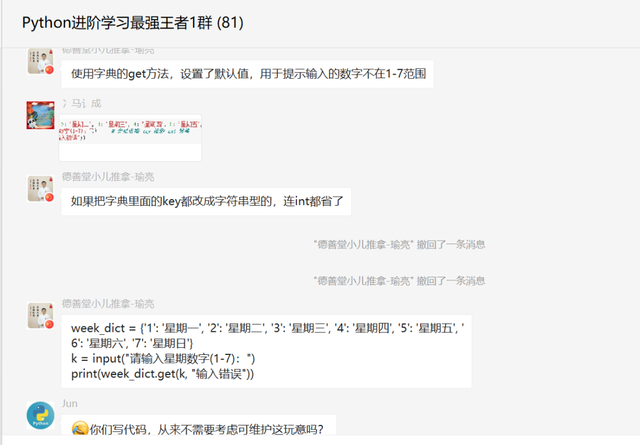# 一、前言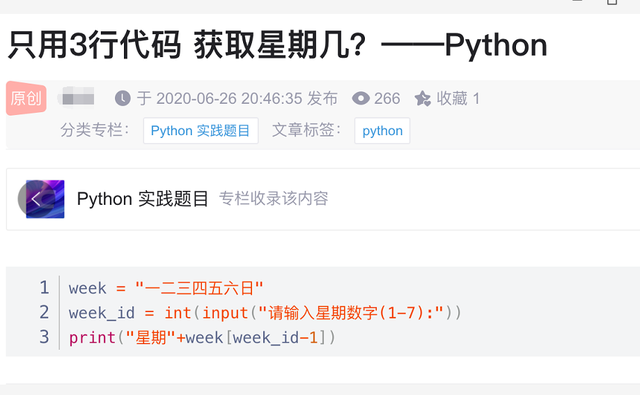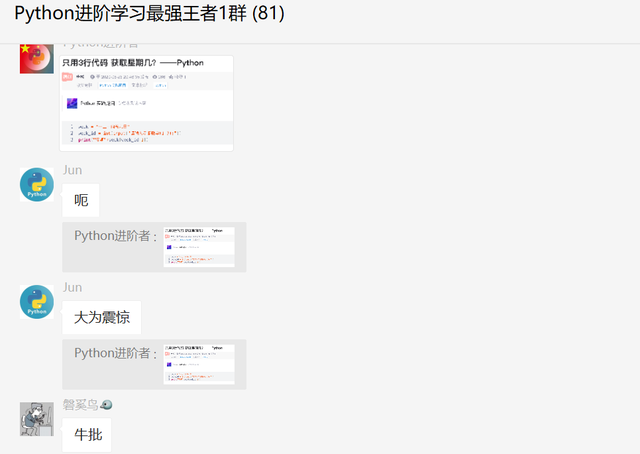# 【冯诚】解答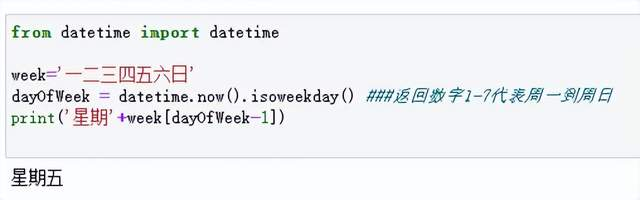# 【月神】解答

``````from datetime import datetime

print(f"星期{list('一二三四五六日')[datetime.today().weekday()]}")
``````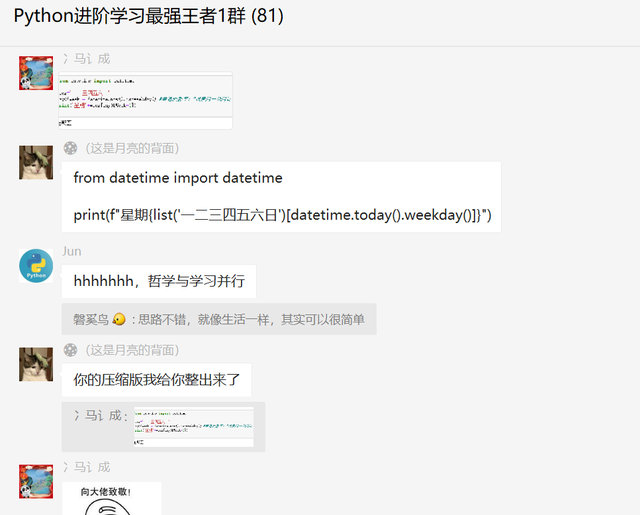# 【瑜亮老师】解答

``````week_dict = {1: '星期一', 2: '星期二', 3: '星期三', 4: '星期四', 5: '星期五', 6: '星期六', 7: '星期日'}
k = int(input("请输入星期数字(1-7)："))     # 此处省略 try 检测 int 转换
print(week_dict.get(k, "输入错误"))
``````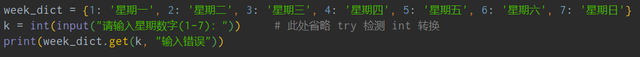``````week_dict = {'1': '星期一', '2': '星期二', '3': '星期三', '4': '星期四', '5': '星期五', '6': '星期六', '7': '星期日'}
k = input("请输入星期数字(1-7)：")
print(week_dict.get(k, "输入错误"))
``````